# Breast Cancer Detection Using Machine Learning

In this article I will show you how to create your very own machine learning python program to detect breast cancer from data. Breast Cancer (BC) is a common cancer for women around the world, and early detection of BC can greatly improve prognosis and survival chances by promoting clinical treatment to patients early. So it’s amazing to be able to possibly help save lives just by using data, python, and machine learning!

If you prefer not to read this article and would like a video representation of it, you can check out the YouTube Video below. It goes through everything in this article with a little more detail, and will help make it easy for you to start programming your own Machine Learning model even if you don’t have the programming language Python installed on your computer. Or you can use both as supplementary materials for learning about Machine Learning !

# Start Programming:

The first thing that I like to do before writing a single line of code is to put in a description in comments of what the code does. This way I can look back on my code and know exactly what it does.

`#Description: This program detects breast cancer, based off of data. `

Now import the packages/libraries to make it easier to write the program.

`#import libraries import numpy as np import pandas as pd import matplotlib.pyplot as plt import seaborn as sns`

Next I will load the data, and print the first 7 rows of data.

NOTE: Each row of data represents a patient that may or may not have cancer.

`#Load the data #from google.colab import files # Use to load data on Google Colab #uploaded = files.upload() # Use to load data on Google Colab df = pd.read_csv('data.csv') df.head(7)`

Explore the data and count the number of rows and columns in the data set. Their are 569 rows of data which means their are 569 patients in this data set, and 33 columns which mean their are 33 features or data points for each patient.

`#Count the number of rows and columns in the data setdf.shape`

Continue exploring the data and get a count of all of the columns that contain empty (NaN, NAN, na) values. Notice none of the columns contain any empty values except the column named ‘Unnamed: 32’ , which contains 569 empty values (the same number of rows in the data set, this tells me this column is completely useless)

`#Count the empty (NaN, NAN, na) values in each columndf.isna().sum()`

Remove the column ‘Unnamed: 32 from the original data set since it adds no value.

`#Drop the column with all missing values (na, NAN, NaN)#NOTE: This drops the column Unnameddf = df.dropna(axis=1)`

Get the new count of the number of rows and columns.

`#Get the new count of the number of rows and colsdf.shape`

Get a count of the number of patients with Malignant (M) cancerous and Benign (B) non-cancerous cells.

`#Get a count of the number of 'M' & 'B' cellsdf['diagnosis'].value_counts()`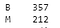# of Cancerous Cells: 212 and # of Non-Cancerous Cells: 357

Visualize the counts, by creating a count plot.

`#Visualize this count sns.countplot(df['diagnosis'],label="Count")`

Look at the data types to see which columns need to be transformed / encoded. I can see from the data types that all of the columns/features are numbers except for the column ‘diagnosis’, which is categorical data represented as an object in python.

`#Look at the data types df.dtypes`

Encode the categorical data. Change the values in the column ‘diagnosis’ from M and B to 1 and 0 respectively, then print the results.

`#Encoding categorical data values (from sklearn.preprocessing import LabelEncoderlabelencoder_Y = LabelEncoder()df.iloc[:,1]= labelencoder_Y.fit_transform(df.iloc[:,1].values)print(labelencoder_Y.fit_transform(df.iloc[:,1].values))`

Create a pair plot. A “pairs plot” is also known as a scatter plot, in which one variable in the same data row is matched with another variable’s value.

`sns.pairplot(df, hue="diagnosis")`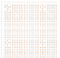Pair plot of all of the columns highlighting the diagnosis points in Orange (1) & Blue (0)

Print the new data set which now has only 32 columns. Print only the first 5 rows.

`df.head(5)`

Get the correlation of the columns.

`#Get the correlation of the columnsdf.corr()`

Visualize the correlation by creating a heat map.

`plt.figure(figsize=(20,20))  sns.heatmap(df.corr(), annot=True, fmt='.0%')`

Now I am done exploring and cleaning the data. I will set up my data for the model by first splitting the data set into a feature data set also known as the independent data set (X), and a target data set also known as the dependent data set (Y).

`X = df.iloc[:, 2:31].values Y = df.iloc[:, 1].values `

Split the data again, but this time into 75% training and 25% testing data sets.

`from sklearn.model_selection import train_test_splitX_train, X_test, Y_train, Y_test = train_test_split(X, Y, test_size = 0.25, random_state = 0)`

Scale the data to bring all features to the same level of magnitude, which means the feature / independent data will be within a specific range for example 0–100 or 0–1.

`#Feature Scalingfrom sklearn.preprocessing import StandardScalersc = StandardScaler()X_train = sc.fit_transform(X_train)X_test = sc.transform(X_test)`

Create a function to hold many different models (e.g. Logistic Regression, Decision Tree Classifier, Random Forest Classifier) to make the classification. These are the models that will detect if a patient has cancer or not. Within this function I will also print the accuracy of each model on the training data.

`def models(X_train,Y_train):    #Using Logistic Regression   from sklearn.linear_model import LogisticRegression  log = LogisticRegression(random_state = 0)  log.fit(X_train, Y_train)    #Using KNeighborsClassifier   from sklearn.neighbors import KNeighborsClassifier  knn = KNeighborsClassifier(n_neighbors = 5, metric = 'minkowski', p = 2)  knn.fit(X_train, Y_train)  #Using SVC linear  from sklearn.svm import SVC  svc_lin = SVC(kernel = 'linear', random_state = 0)  svc_lin.fit(X_train, Y_train)  #Using SVC rbf  from sklearn.svm import SVC  svc_rbf = SVC(kernel = 'rbf', random_state = 0)  svc_rbf.fit(X_train, Y_train)  #Using GaussianNB   from sklearn.naive_bayes import GaussianNB  gauss = GaussianNB()  gauss.fit(X_train, Y_train)  #Using DecisionTreeClassifier   from sklearn.tree import DecisionTreeClassifier  tree = DecisionTreeClassifier(criterion = 'entropy', random_state = 0)  tree.fit(X_train, Y_train)  #Using RandomForestClassifier method of ensemble class to use Random Forest Classification algorithm  from sklearn.ensemble import RandomForestClassifier  forest = RandomForestClassifier(n_estimators = 10, criterion = 'entropy', random_state = 0)  forest.fit(X_train, Y_train)    #print model accuracy on the training data.  print('Logistic Regression Training Accuracy:', log.score(X_train, Y_train))  print('K Nearest Neighbor Training Accuracy:', knn.score(X_train, Y_train))  print('Support Vector Machine (Linear Classifier) Training Accuracy:', svc_lin.score(X_train, Y_train))  print('Support Vector Machine (RBF Classifier) Training Accuracy:', svc_rbf.score(X_train, Y_train))  print('Gaussian Naive Bayes Training Accuracy:', gauss.score(X_train, Y_train))  print('Decision Tree Classifier Training Accuracy:', tree.score(X_train, Y_train))  print('Random Forest Classifier Training Accuracy:', forest.score(X_train, Y_train))    return log, knn, svc_lin, svc_rbf, gauss, tree, forest`

Create the model that contains all of the models, and look at the accuracy score on the training data for each model to classify if a patient has cancer or not.

`model = models(X_train,Y_train)`

Show the confusion matrix and the accuracy of the models on the test data. The confusion matrix tells us how many patients each model misdiagnosed (number of patients with cancer that were misdiagnosed as not having cancer a.k.a false negative, and the number of patients who did not have cancer that were misdiagnosed with having cancer a.k.a false positive) and the number of correct diagnosis, the true positives and true negatives.

False Positive (FP) = A test result which incorrectly indicates that a particular condition or attribute is present.

True Positive (TP) = Sensitivity (also called the true positive rate, or probability of detection in some fields) measures the proportion of actual positives that are correctly identified as such.

True Negative (TN) = Specificity (also called the true negative rate) measures the proportion of actual negatives that are correctly identified as such.

False Negative (FN) = A test result that indicates that a condition does not hold, while in fact it does. For example a test result that indicates a person does not have cancer when the person actually does have it

`from sklearn.metrics import confusion_matrixfor i in range(len(model)):  cm = confusion_matrix(Y_test, model[i].predict(X_test))    TN = cm  TP = cm  FN = cm  FP = cm    print(cm)  print('Model[{}] Testing Accuracy = "{}!"'.format(i,  (TP + TN) / (TP + TN + FN + FP)))  print()# Print a new line`

Other ways to get metrics on the model to see how well each one performed.

`#Show other ways to get the classification accuracy & other metrics from sklearn.metrics import classification_reportfrom sklearn.metrics import accuracy_scorefor i in range(len(model)):  print('Model ',i)  #Check precision, recall, f1-score  print( classification_report(Y_test, model[i].predict(X_test)) )  #Another way to get the models accuracy on the test data  print( accuracy_score(Y_test, model[i].predict(X_test)))  print()#Print a new line`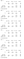Sample of the models from 1–6 performance metrics on test data

From the accuracy and metrics above, the model that performed the best on the test data was the Random Forest Classifier with an accuracy score of about 96.5%. So I will choose that model to detect cancer cells in patients. Make the prediction/classification on the test data and show both the Random Forest Classifier model classification/prediction and the actual values of the patient that shows rather or not they have cancer.

I notice the model, misdiagnosed a few patients as having cancer when they didn’t and it misdiagnosed patients that did have cancer as not having cancer. Although this model is good, when dealing with the lives of others I want this model to be better and get it’s accuracy as close to 100% as possible or at least as good as if not better than doctors. So a little more tuning of each of the models is necessary.

`#Print Prediction of Random Forest Classifier modelpred = model.predict(X_test)print(pred)#Print a spaceprint()#Print the actual valuesprint(Y_test)`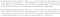Top: Decision Tree Classifier prediction, Bottom: The actual classification of the patients

That is it, you are done creating your breast detection program to predict if a patient has cancer or not! Again if you want, you can watch and listen to me explain all of the code on my YouTube video.

If you are interested in reading more on machine learning to immediately get started with problems and examples then I strongly recommend you check out Hands-On Machine Learning with Scikit-Learn and TensorFlow: Concepts, Tools, and Techniques to Build Intelligent Systems. It is a great book for helping beginners learn how to write machine learning programs, and understanding machine learning concepts.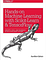Hands-On Machine Learning with Scikit-Learn and TensorFlow: Concepts, Tools, and Techniques to Build Intelligent Systems